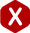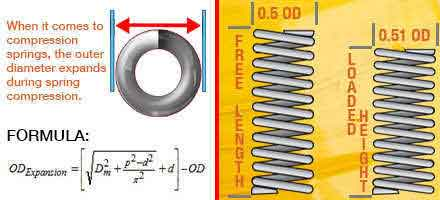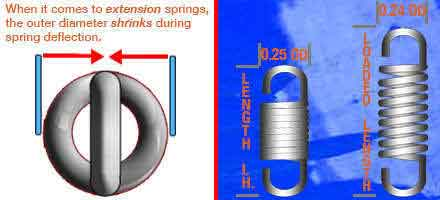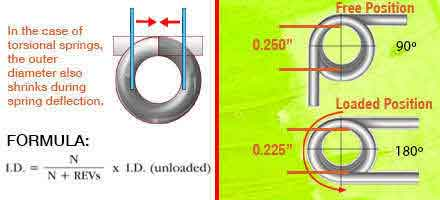<# Spring Diameter Change

#### Definition: The change in size of a spring’s diameter during deflection.

When a coil spring is deflected, whether it is a compression, extension or torsion spring, its diameter is affected. In some cases the diameter will shrink while in other cases it will expand. Below are the examples of the spring diameter change of compression, tension and torsional springs.

#### Compression Spring Diameter Change

In the case of a compression spring, its diameter expands when such compression spring has deflected. Because of the way its coils are shaped with pitch in between them, they have memory in them. This means that when a compression spring is deformed, the memory in the coils stores an amount of energy and is “programmed” to expand slightly once the load which made it compress is removed. This is something you must take into account when your spring will be going inside a hole. The formula to calculate this factor is provided to the right.

Expanded OD = [{Dm2+ [(p2– d2)÷π2]}1/2+ d] – OD
• Dm = Mean Diameter
• p = Pitch
• d = Wire Diameter
• OD = Outer Diameter
• π = Pi (3.14)
• Expanded OD = Expanded Outer Diameter

#### Diagram FormulaThe numbers on this example should not be used for reference and are only for theoretical purposes.

#### Extension Spring Diameter Change

In the case of an extension spring, it does the exact opposite of what a compression spring does. A tension spring’s body is pulled, not compressed. Therefore, an extension spring’s diameter shrinks as the spring’s coils are being extended. When it comes to an extension spring, it is rarely going to go over a shaft so this shouldn’t be a problem. The example to the right shows how an extension spring’s diameter changes after deflection.

#### Diagram ExampleThe numbers on this example should not be used for reference and are only for theoretical purposes.

#### Torsion Spring Diameter Change

When it comes to a torsional spring, its deflection is rotational. This means that when one of the torsion spring’s legs are in motion, the torsion spring’s body is being tightened. Therefore, since the spring is being torqued and tightened, the torsional spring diameter shrinks. Because torsion springs will usually be placed over a shaft, you want to make sure that the spring’s inner diameter isn’t gonna shrink to the point where it grips (bites down) on the mandrel or shaft. An example of torsion spring deflection is shown to the right as well.

Torqued ID = N ÷ (N + REVs) x ID
• N = Number of Coils
• REVs = Leg Revolutions
• Torqued ID = Torqued Inner Diameter
• ID = Unloaded Inner Diameter

#### Example and FormulaThe numbers on this example should not be used for reference and are only for theoretical purposes.